## Note

This is a collection of my undergraduate assignments that I translated to English myself in the Basic Electrical Power Engineering course. This assignment has never been published anywhere and I, as the author and copyright holder, license this assignment customized CC-BY-SA where anyone can share, copy, republish, and sell on condition to state my name as the author and notify that the original and open version available here.

## General Electric Energy Supply Process

### Electrical Conversion

Conversion in the field of electric power is the conversion from other energy to electrical energy. This conversion is usually used to generate electricity, producing this electricity is called a generator, in other words as a producer. Here are example sources of electrical energy: Hydroelectric Power Plant, Steam Power Plant, Nuclear Power Plant, Gas Power Plant, and Diesel Power Plant. Other generators include: Solar Power, Wind Power, Chemical Reactions, Geothermal Energy and others.

Nuclear Energy etc → Reactor Converter etc → Alternate → Transformer Step Up; Reactor Converter etc → Generate

### Electric Transmission

Electric transmission is the process of sending electricity. The delivery goes through the power grid.

Step-Up Transformer → High Voltage Network → Step-Down Transformer → Medium Voltage Network → Factory

### Electricity Distribution

Electricity distribution is the process of sharing electricity to consumers.

Medium Voltage Network → Step-Down Transformer → Low Voltage Network → Consumer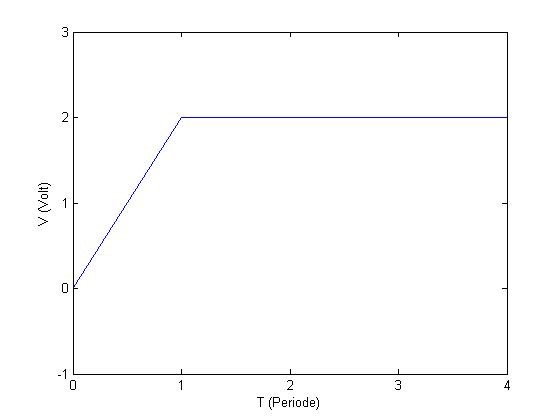DC (Direct Current) electric graph, V(t) = 2 volt, V(0) = 0 volt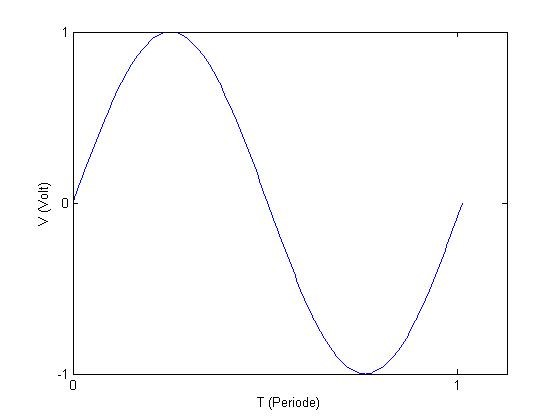AC (Alternating Current) electric graph, V(t) = sin(wt)

## Basic Theories

### Ohm Law

Ohm law: V=IR, V = voltage (volt), I = current (ampere), R = resistance (ohm).

### Inverter, Rectifier, UPS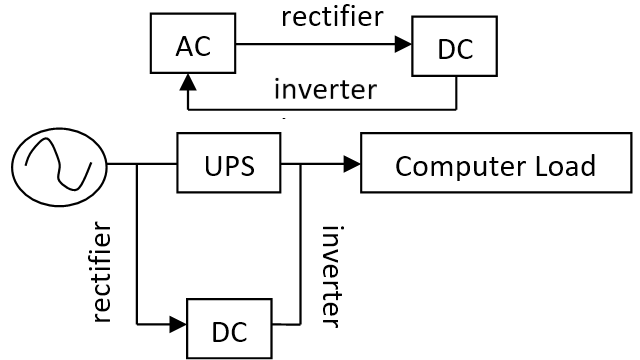AC (alternating current) electricity to DC, using a rectifier. DC (direct current) electricity to AC, using an inverter. If the electricity suddenly goes out, it can damage the computer, hardware and software (data). To anticipate this, a UPS was created. The UPS supplies AC power to the computer as well as stores electricity in DC form. If the power suddenly goes out, the computer will use the UPS stored power, at least giving it time to store data and shut down the computer safely.

### Transformator

Transformers are devices for changing the voltage. The step-down transformer lowers the voltage while the step-up transformer increases the voltage.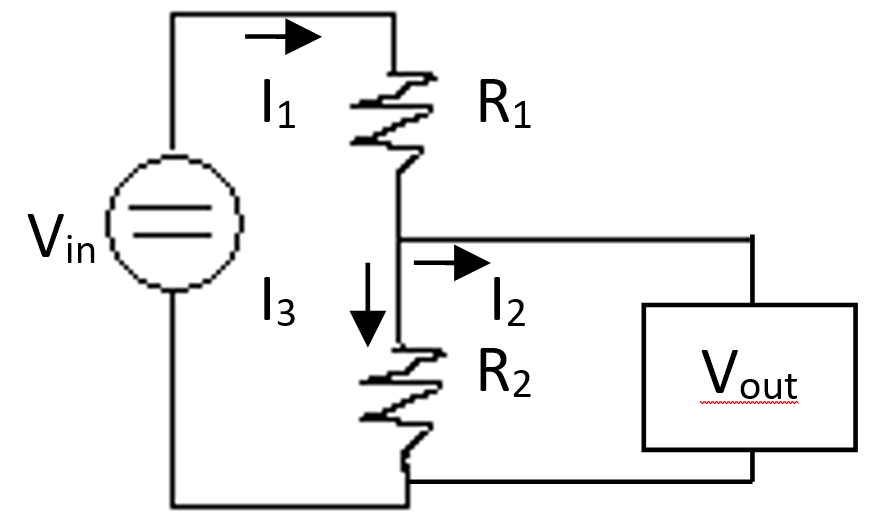Example DC step-down transformer, DC step-up transformer when the circuit is reversed. Vin = V1+V2 = I1 R1+I3 R2. I1 = I2+I3. Vout = V2 = Vin-V1.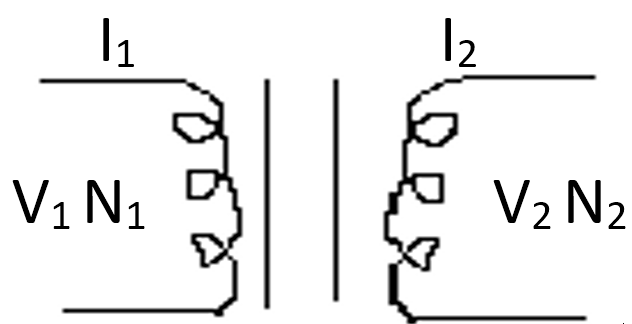AC Transformator. V1/V2 = N1/N2 = I2/I1.

### Magnetic Field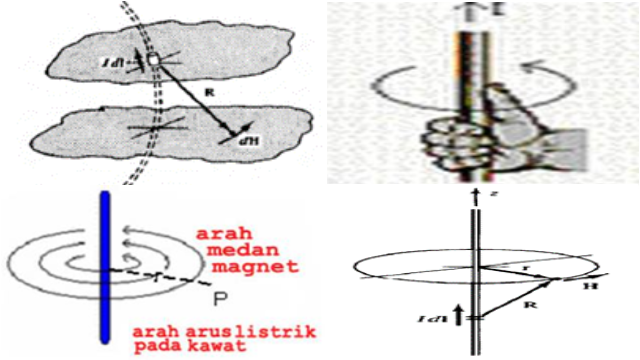If there is a current flowing I towards dl (long) then the magnetic field strength H.

dH = (IdI x aR)/(4πR2)(A/m), H = ∮(IdI x aR)/(4πR2)

I = current (A), dl = length, aR = vector, R = vector length.

Magnetic Field Flux

B = dΦ/ds = μH, Φ = ∮Bds

B = magnetic field flux density (weber/area), Φ=magnetic field flux (weber), ds = area (m2), H = magnetic field strength (A/m)

### Direct Current Generator Working Principle

The working principle of a direct current generator is based on Faraday's law: e = -N dΦ/dt

Where, N: number of turns, Φ: magnetic flux, e: induced voltage, emf (electromotive force).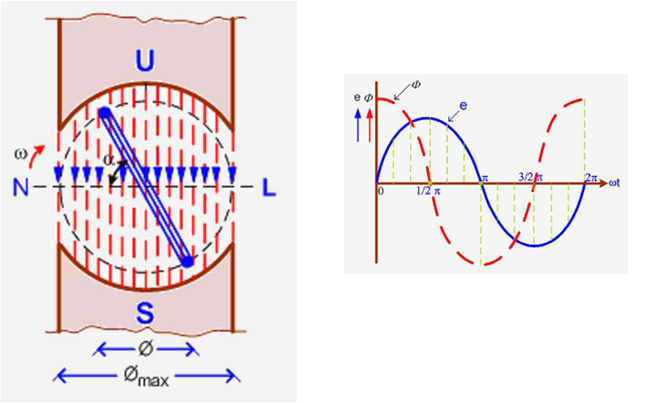The process of forming an emf on the side of the generator coil.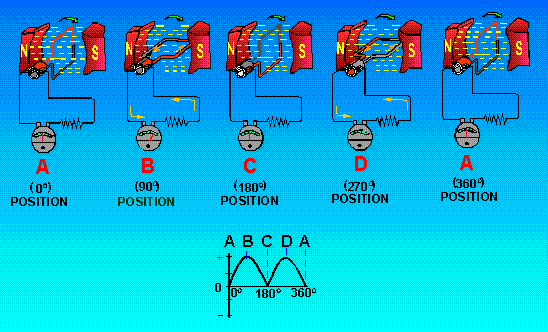The resulting voltage is an alternating current. Furthermore, the current will be rectified by the commutator.

The commutator functions as a switch. The commutator is in the form of a split ring attached to the end of the anchor. When the anchor rotates, the ring will rotate. When the coil has rotated half a turn, the brush will close the ring gap so that the voltage becomes zero. Because the ring continues to rotate, the gap will open again and create tension again. If the voltage period is the same as the ring rotation period, the voltage that arises is the full wave direct current voltage.

### Types of Direct Current Generators Viewed from its Field Winding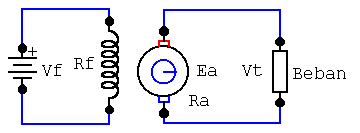Separated gain DC generator. Vf = If Rf, Ea = Vt + Ia Ra

#### Self-Strengthening Generator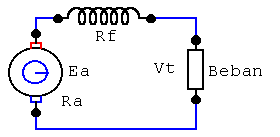Series. Vt = Ia Ra, Ea = Ia (Ra + Rf) + Vt + < Vsi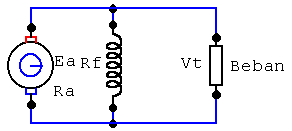Shunt. Vt = If Rf, Ea = Ia Ra + Vt + < Vsi

#### Compound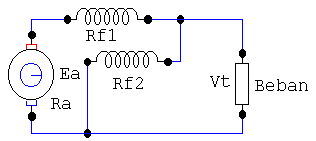Long. Ia = If1 = IL + If2, Ea = Vt + Ia(Ra + Rf1) + < Vsi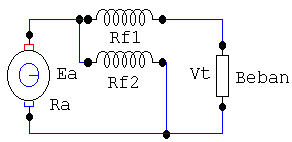Short. Ia = If1 + If2 = IL + If2, Ea = Vt + ILRf1 + IaRa + < Vsi

## Some Exercises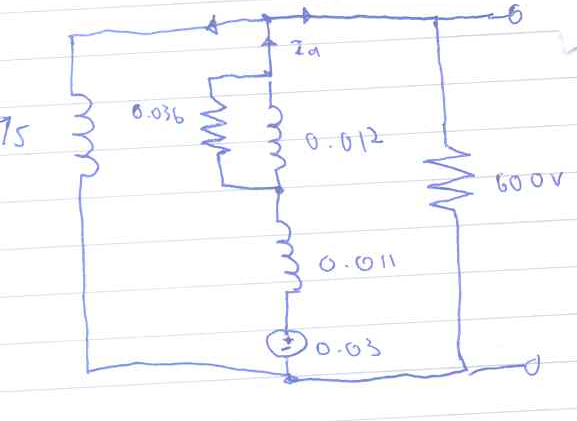1. A long compound DC generator provides 300 kW of power at a terminal voltage of 600V. The parallel field resistance is 75 the armature resistance, the brush resistance is 0.03, the field winding commutation resistance is 0.011Ω the series field resistance is 0.012Ω, the divertor resistance is 0.036. When the generator is fully loaded calculate the voltage and power generated by the armature winding!

Ish = 600V/75Ω = 8A, I = 300000W/600V = 500A, Ia = Ish+I = 8A+500A = 508A

∆Vtotal = ∆V+∆Va = Ia R+Ia Ra = Ia (R+Ra) = 508A(0.03Ω+0.02Ω) = 25.4V

emfV = V+∆Vtotal = 600V+20.4V = 625.4V

emfP = (emfV)(Ia) = (625.4V)(508A) = 317703.2W

2. Transformer 1ɸ is given a 50 Hz supply step down 2200 V → 250 V with an area of 36 cm2 and a flux density of 6 wb/m2, look for the primary and secondary windings.

Known:

f = 50 Hz

E1 = 2200 V

E2 = 250 V

A = 0.0036 m2

Bm = 6 wb/m2

N1 and N2

Φm = (Bm)(A)=(6 wb/m2)(0.0036 m2) = 0.0216 wb

E = (4.44)(f)(N)(Φm) = (4.44)(f)(N)(Bm)(A)

Primary Winding = E1/((4.44)(f)(Φm)) = 2200/((4.44)(50 Hz)(0.0216 wb)) = 458.79

Secondary Winding = E2/((4.44)(f)(Φm)) = 250/((4.44)(50 Hz)(0.0216 wb)) = 52.135

3. The power of the transformer 1ɸ is 25 KVI, the primary winding is 500, the secondary winding is 50, the primary winding is connected to a voltage of 3000 V with a frequency of 50 Hz. Find the load currents in the primary and secondary winding, secondary emf and maximum flux. (ignore the drop voltage)

Known

P = 25 VI

N1 = 500

N2 = 50

E1 = 3000 V

f = 50 Hz

I1, I2, E2 and Φm

E = (4.44)(f)(N)(Φm) = (4.44)(f)(N)(Bm)(A)

Φm = (4.44)(f)(N1)/E1 = (4.44)(50 Hz)(500)/(3000 V) = 37 wb

E2/E1 = (4.44)(f)(N2)(Φm)/(4.44)(f)(N1)(Φm) = N2/N1

E2=N2/N1 E1 = 500/50 3000 V = 30000V

P=(V)(I)

I1 = P/E1 = (25 W)/(3000 V) = 8.3mA

I2 = P/E2 = (25 W)/(30000 V) = 0.83mA